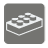SendGrip Spam Report
Undefined
English

( Describe task in general )

Sum two passed ints( Explain input and output values )

Input: Two arguments. Both are int

Output: Int.

( Put some usage examples )

Example:

```sum_two(2, 3) == 5
sum_two(5, 7) == 12
```

How it is used: (Here you can explain how it can be used in development and what is usage of this.)

Precondition: Constraints for Input